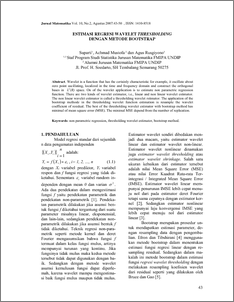# ESTIMASI REGRESI WAVELET THRESHOLDING DENGAN METODE BOOTSTRAP

Suparti, Suparti and Mustofa, Achmad and Rusgiyono, Agus (2007) ESTIMASI REGRESI WAVELET THRESHOLDING DENGAN METODE BOOTSTRAP. JURNAL MATEMATIKA KOMPUTER, 10 (2). pp. 43-50. ISSN 1410-8518Preview
PDF
213Kb

## Abstract

Wavelet is a function that has the certainly characteristic for example, it oscillate about zero point ascillating, localized in the time and frequency domain and construct the orthogonal bases in L2(R) space. On of the wavelet application is to estimate non parametric regression function. There are two kinds of wavelet estimator, i.e., linear and non linear wavelet estimator. The non linear wavelet estimator is called a thresholding wavelet rstimator. The application of the bootstrap methode in the thresholding wavelet function estimation is resample the wavelet coefficient of residual. The best of the thresholding wavelet estimator with bootstrap method has minimal of mean square error (MSE). The minimal MSE depend from the number of replication.

Item Type: Article non parametric regression, thresholding wavelet estimator, bootstrap method. Q Science > QA Mathematics Faculty of Science and Mathematics > Department of Mathematics 1854 INVALID USER 30 Nov 2009 10:27 08 Dec 2009 09:18

Repository Staff Only: item control page